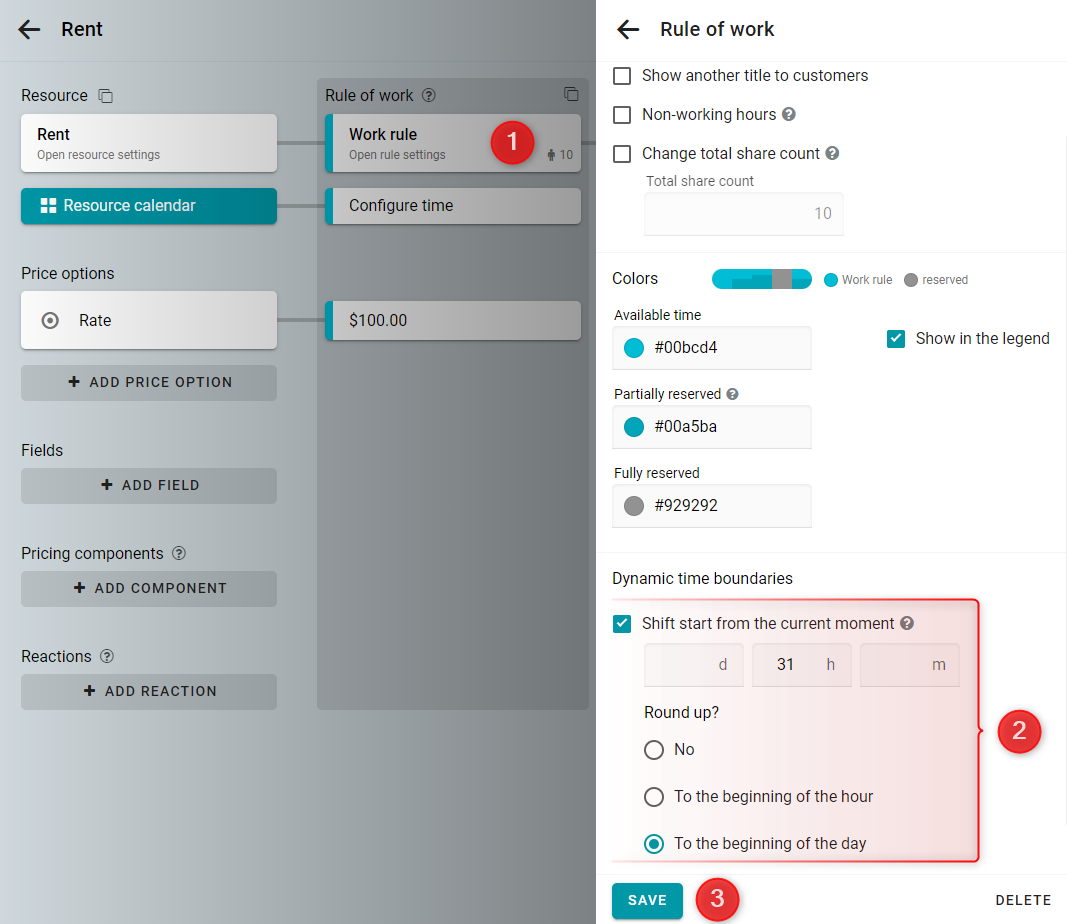arrow_back_iosQuestions
help

We close reservations for tomorrow at exactly 17:00. How can we configure it?

check_circle
You can implement this configuration using dynamic time boundaries. At 17:00, the start should be shifted to the day after tomorrow. Let's calculate how many hours you need to shift the start:
(24 - 17) + 24 = 7 + 24 = 31.
Let's configure this for hourly rental.
Open the rule of work settings. Set the parameter Shift start from the current moment to 31 h. Then set round up to the beginning of the day. Save it.Now at 16:00, the time will first shift to 23:00 tomorrow and then round up to the beginning of tomorrow.
And at 18:00, the time will first shift to 1:00 the day after tomorrow and then round up to the beginning of the day after tomorrow.